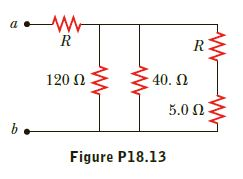Question
1 views

The resistance between
terminals a and b in Figure
P18.13 is 75 Ω. If the resistors
labeled R have the
same value, determine R.help_outlineImage TranscriptioncloseR 120 N 40. N 5.0 Ω. Figure P18.13 fullscreen
check_circle

Step 1

Given,

The resistance between the two points a and b is 75.0 Ω.

Step 2

The resistance R and R5.00Ω are in series. Thus, the equivalent resistance is

Step 3

Figure.1 shows the equi...

### Want to see the full answer?

See Solution

#### Want to see this answer and more?

Solutions are written by subject experts who are available 24/7. Questions are typically answered within 1 hour.*

See Solution
*Response times may vary by subject and question.
Tagged in

### Current Electricity